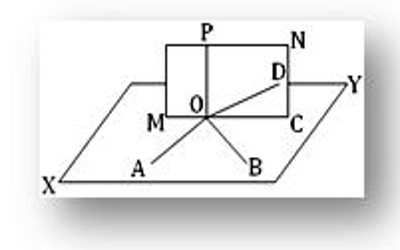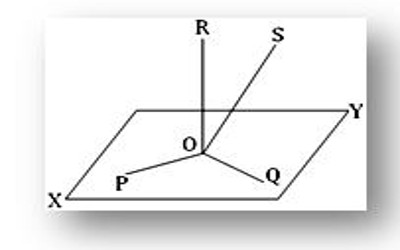Theorem on Co-planar

A set of points, lines, line segments, rays or any other geometrical shapes that lie on the same plane are said to be Coplanar. In geometry, a set of points in space are coplanar if there exists a geometric plane that contains them all. For example, three points are always coplanar, and if the points are distinct and non-collinear, the plane they determine is unique.

Theorem: All straight lines drawn a perpendicular to a straight line at a given point on it are co-planar.

Let OP be the given straight line and each of the straight lines OA, OB and OC be perpendicular to OP at O.

We are to prove that the straight lines OA, OB, and OC are co-planar.Construction: We know that one and only one plane can be drawn through two intersecting straight lines. Let XY be the plane through the intersecting straight lines OA and OB and MN be the plane through the intersecting straight lines OC and OP. let us assume that these two planes intersect in the straight line OD.

Proof: Since OP is perpendicular to both OA and OB at their point of intersection O, hence OP is perpendicular to the plane XY. Now, OD is the line of intersection of the planes XY and MN; hence, OD lies in the plane XY and it meets OP at O. therefore, OP is perpendicular to OD. Again, OP is perpendicular to OC (given proposition). Thus, we see that straight lines OP, OC and OD all lie in one plane (i.e., in the plane MN) and each of OC and OD is perpendicular to OP at the same point O. evidently, this is impossible unless OC and OD coincide. Therefore, OC lies in the XY plane (since OC and OD represent the same line and OD lies in the XY plane).

Therefore, The straight line OA, OB and OC all lie in the XY plane i.e., they are co-planar.

Similarly, it can be shown that any straight line drawn a perpendicular to OP at O lie in the XY plane.

Therefore, all straight lines drawn a perpendicular to OP at Q are co-planar.

Examples: Can there be more than three straight lines perpendicular to each other at a point in three-dimensional space? Justify your answer.If possible, let four straight lines OP, OQ, OR and OS be perpendicular to each other at the point O in three-dimensional spaces. Let XY be the plane through the intersecting straight lines OP and OQ. Since OR is perpendicular to both OP and OQ at their point of intersection O, hence OR is perpendicular to the XY plane at O. Again, OS is also perpendicular to each of OP and OQ at the point O. Hence, OS is also perpendicular to the XY plane at O.

Thus, we see that each of OR and OS is perpendicular to the XY plane at the same point O. Evidently, this is impossible unless OR and OS coincide. Therefore, it is impossible to have more than three straight lines perpendicular to each other at a point in three-dimensional spaces.

Information Source: